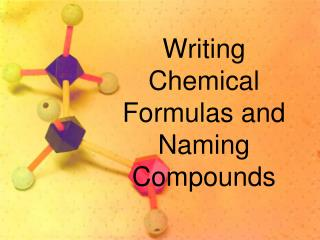Download PresentationWriting Chemical Formulas and Naming Compounds

# Writing Chemical Formulas and Naming Compounds - PowerPoint PPT PresentationDownload Presentation## Writing Chemical Formulas and Naming Compounds

- - - - - - - - - - - - - - - - - - - - - - - - - - - E N D - - - - - - - - - - - - - - - - - - - - - - - - - - -
##### Presentation Transcript

1. WritingChemical Formulas and Naming Compounds

2. Vanadium (III) peroxide write a correct formula

3. Write the Correct Formula V3+ O22- V2(O2)3 2 3 Crisscross and use parentheses for the more than one polyatomic ions, but NOT for the monatomic ion

4. Cadmium phosphide write a correct formula

5. Write the Correct Formula Cd2+ P3- Cd3P2 3 2 Crisscross and NO parentheses because there are NO polyatomic ions

6. Chromium (III) chloride write a correct formula

7. Write the Correct Formula Cr3+Cl- CrCl3 1 3 Crisscross and NO parentheses because there are NO polyatomic ions

8. Ammonium dichromate write a correct formula

9. Write the Correct Formula NH4+ Cr2O72- (NH4)2Cr2O7 2 1 Crisscross and use parentheses for the more than one polyatomic ions, but NOT for the single polyatomic ion

10. Aluminum arsenate write a correct formula

11. Write the Correct Formula Al3+ AsO43- AlAsO4 No need to crisscross because the charges are balanced; NO parentheses for monatomic and single polyatomic ions

12. Magnesium sulfide write a correct formula

13. Write the Correct Formula Mg2+ S2- MgS No need to crisscross because the charges are balanced; NO parentheses for the monatomic ions

14. Iron (II) acetate write a correct formula

15. Write the Correct Formula Fe2+ CH3COO- Fe(CH3COO)2 1 2 Crisscross and use parentheses for the more than one polyatomic ions, but NOT for the monatomic ion

16. Ammonium chromate write a correct formula

17. Write the Correct Formula NH4+ CrO42- (NH4)2CrO4 2 1 Crisscross and use parentheses for the more than one polyatomic ions, but NOT for the single polyatomic ion

18. Zinc hydrogensulfate write a correct formula

19. Write the Correct Formula Zn2+ HSO4- Zn(HSO4)2 1 2 Crisscross and use parentheses for the more than one polyatomic ions, but NOT for the monatomic ion

20. Tin (IV) hypochlorite write a correct formula

21. Write the Correct Formula Sn4+ClO- Sn(ClO)4 1 4 Crisscross and use parentheses for the more than one polyatomic ions, but NOT for the monatomic ion

22. Lead (IV) sulfate write a correct formula

23. Write the Correct Formula Pb4+ SO42- Pb(SO4)2 2 4 Crisscross, SIMPLIFY, and use parentheses for the more than one polyatomic ions, but NOT for the monatomic ion

24. Copper (II) dihydrogenphosphate write a correct formula

25. Write the Correct Formula Cu2+ H2PO4- Cu(H2PO4)2 1 2 Crisscross and use parentheses for the more than one polyatomic ions, but NOT for the monatomic ion

26. Barium permanganate write a correct formula

27. Write the Correct Formula Ba2+ MnO4- Ba(MnO4)2 1 2 Crisscross and use parentheses for the more than one polyatomic ions, but NOT for the monatomic ion

28. Calcium chlorate write a correct formula

29. Write the Correct Formula Ca2+ ClO3- Ca(ClO3)2 1 2 Crisscross and use parentheses for the more than one polyatomic ions, but NOT for the monatomic ion

30. Ammonium oxide write a correct formula

31. Write the Correct Formula NH4+ O2- (NH4)2O 2 1 Crisscross and use parentheses for the more than one polyatomic ions, but NOT for the monatomic ion

32. Silver chlorite write a correct formula

33. Write the Correct Formula Ag+ ClO2- AgClO2 No need to crisscross because the charges are balanced; NO parentheses for monatomic and single polyatomic ions

34. Mercury (II) iodide write a correct formula

35. Write the Correct Formula Hg2+I- Hgl2 1 2 Crisscross and NO parentheses because there are NO polyatomic ions

36. Beryllium carbonate write a correct formula

37. Write the Correct Formula Be2+ CO32- BeCO3 No need to crisscross because the charges are balanced; NO parentheses for monatomic and single polyatomic ions

38. Sodium phosphate write a correct formula

39. Write the Correct Formula Na+ PO43- Na3PO4 3 1 Crisscross and NO parentheses for monatomic and single polyatomic ions

40. Iron (III) bromide write a correct formula

41. Write the Correct Formula Fe3+ Br- FeBr3 1 3 Crisscross and NO parentheses because there are NO polyatomic ions

42. Potassium nitrate write a correct formula

43. Write the Correct Formula K+ NO3- KNO3 No need to crisscross because the charges are balanced; NO parentheses for monatomic and single polyatomic ions

44. Lithium nitride write a correct formula

45. Write the Correct Formula Li+ N3- Li3N 3 1 Crisscross and NO parentheses because there are NO polyatomic ions

46. Lead (II) hydroxide write a correct formula

47. Write the Correct Formula Pb2+ OH- Pb(OH)2 1 2 Crisscross and use parentheses for the more than one polyatomic ions, but NOT for the monatomic ion

48. Niobium (V) perchlorate write a correct formula

49. Write the Correct Formula Nb5+ ClO4- Nb(ClO4)5 1 5 Crisscross and use parentheses for the more than one polyatomic ions, but NOT for the monatomic ion

50. Cobalt (III) hydrogenphosphate write a correct formula